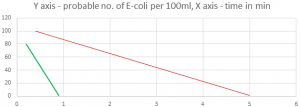Q.1
##### When turbidity in water increases, the efficiency of chlorine?
• a) Decreases
• b) Increases
• c) Slightly increases
• d) Remains constant
Q.2
##### The presence of metallic compounds in water _______ the efficiency of chlorine.
• a) Decreases
• b) Increases
• c) Slightly decreases
• d) Remains constant
Q.3
##### The amount of hypochlorous acid formed when pH of water is 8 is _______________
• a) 95% of total free chlorine
• b) 80% of total free chlorine
• c) 30% of total free chlorine
• d) 5% of total free chlorine
Q.4
##### Which type of chlorine is represented by the green line as shown in the following figure?• a) Free residual chlorine
• b) Ammonia chlorine
• c) Combined residual chlorine
• d) Residual chloramine
Q.5
##### Which type of chlorine is represented by the red line as shown in the following figure?• a) Free residual chlorine
• b) Ammonia chlorine
• c) Combined residual chlorine
• d) Residual chloramine
Q.6
##### The efficiency of chlorine increase by ______________
• a) Presence of metallic compounds
• b) When the pH of the water increases
• c) When the pH of the water decreases
• d) Turbid water
Q.7
##### The contact time for disinfection by free chlorine is ______________
• a) 20 minutes
• b) 30 minutes
• c) 40 minutes
• d) 60minutes
Q.8
##### The contact time for disinfection by combined chlorine is ______________
• a) 20 minutes
• b) 30 minutes
• c) 40 minutes
• d) 60 minutes